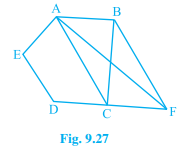In Fig. 9.27, ABCDE is a pentagon. A line through B parallel to AC meets DC produced at F. Show that (i) ar (ACB) = ar (ACF) (ii) ar (AEDF) = ar (ABCDE)

# In Fig. 9.27, ABCDE is a pentagon. A line through B parallel to AC meets DC produced at F. Show that (i) ar (ACB) = ar (ACF) (ii) ar (AEDF) = ar (ABCDE)Register to Get Free Mock Test and Study Material

+91

Live ClassesRecorded ClassesTest SeriesSelf Learning

Verify OTP Code (required)

### Solution:

i) ΔACB and ΔACF are lying on the same base AC and are existing between the same parallels AC and BF.

According to Theorem 9.2: Two triangles on the same base (or equal bases) and between the same parallels are equal in area.

Therefore, ar (ΔACB) = ar (ΔACF)

ii) It can be observed that

ar (ΔACB) = ar (ΔACF)

Adding area (ACDE) on both the sides.

Area (ΔACB) + Area (ACDE) = Area (ΔACF) + Area (ACDE)

Area (ABCDE) = Area (AEDF)

Hence proved.

## Related content

 Distance Formula Perimeter of Rectangle Area of Square Area of Isosceles Triangle Pythagoras Theorem Triangle Formulae Volume of Cylinder Perimeter of Triangle Formula Area Formulae Volume Formulae